# Please provide a list of all the shapes in tabular format having lines of symmetry, centre of rotation, order of rotational symmetry and angle of rotation?

Triangles

A Triangle can have 3, or 1 or no lines of symmetry: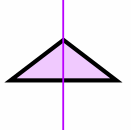Equilateral Triangle(all sides equal, all angles equal)Isosceles Triangle(two sides equal, two angles equal)Scalene Triangle(no sides equal, no angles equal)3 Lines of Symmetry1 Line of SymmetryNo Lines of SymmetryQuadrilaterals

Different types of Quadrilaterals (a 4-sided plane shape):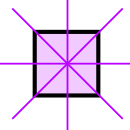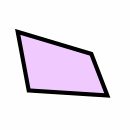Square(all sides equal, all angles 90)Rectangle(opposite sides equal, all angles 90)Irregular Quadrilateral4 Lines of Symmetry2 Lines of SymmetryNo Lines of Symmetry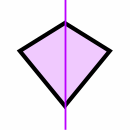KiteRhombus(all sides equal length)1 Line of Symmetry2 Lines of SymmetryRegular Polygons

A regular polygon has all sides equal, and all angles equal:An Equilateral Triangle (3 sides) has 3 Lines of SymmetryA Square (4 sides) has 4 Lines of Symmetry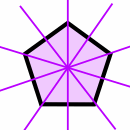A Regular Pentagon (5 sides) has 5 Lines of Symmetry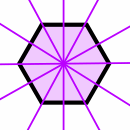A Regular Hexagon (6 sides) has 6 Lines of Symmetry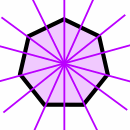A Regular Heptagon (7 sides) has 7 Lines of Symmetry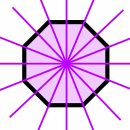A Regular Octagon (8 sides) has 8 Lines of Symmetry

And the pattern continues:

• A regular polygon of 9 sides has 9 Lines of Symmetry
• A regular polygon of 10 sides has 10 Lines of Symmetry
• ...
• A regular polygon of "n" sides has "n" Lines of Symmetry

Circle

A line (drawn at any angle) that goes through its center is a Line of Symmetry.

So a Circle has infinite Lines of Symmetry.

thumbssss up plzzzzzz

• 0
Triangles

A Triangle can have 3, or 1 or no lines of symmetry:Equilateral Triangle(all sides equal, all angles equal)Isosceles Triangle(two sides equal, two angles equal)Scalene Triangle(no sides equal, no angles equal)3 Lines of Symmetry1 Line of SymmetryNo Lines of SymmetryQuadrilaterals

Different types of Quadrilaterals (a 4-sided plane shape):Square(all sides equal, all angles 90)Rectangle(opposite sides equal, all angles 90)Irregular Quadrilateral4 Lines of Symmetry2 Lines of SymmetryNo Lines of SymmetryKiteRhombus(all sides equal length)1 Line of Symmetry2 Lines of SymmetryRegular Polygons

A regular polygon has all sides equal, and all angles equal:An Equilateral Triangle (3 sides) has 3 Lines of SymmetryA Square (4 sides) has 4 Lines of SymmetryA Regular Pentagon (5 sides) has 5 Lines of SymmetryA Regular Hexagon (6 sides) has 6 Lines of SymmetryA Regular Heptagon (7 sides) has 7 Lines of SymmetryA Regular Octagon (8 sides) has 8 Lines of Symmetry

And the pattern continues:

• A regular polygon of 9 sides has 9 Lines of Symmetry
• A regular polygon of 10 sides has 10 Lines of Symmetry
• ...
• A regular polygon of "n" sides has "n" Lines of Symmetry

Circle

A line (drawn at any angle) that goes through its center is a Line of Symmetry.

So a Circle has infinite Lines of Symmetry.

• 1

i cant give you in a tabular form but this will help u a lot

• 0

Triangles

A Triangle can have 3, or 1 or no lines of symmetry:

Equilateral Triangle(all sides equal, all angles equal)Isosceles Triangle(two sides equal, two angles equal)Scalene Triangle(no sides equal, no angles equal)3 Lines of Symmetry1 Line of SymmetryNo Lines of SymmetryQuadrilaterals

Different types of Quadrilaterals (a 4-sided plane shape):

Square(all sides equal, all angles 90)Rectangle(opposite sides equal, all angles 90)Irregular Quadrilateral4 Lines of Symmetry2 Lines of SymmetryNo Lines of Symmetry

KiteRhombus(all sides equal length)1 Line of Symmetry2 Lines of SymmetryRegular Polygons

A regular polygon has all sides equal, and all angles equal:

An Equilateral Triangle (3 sides) has 3 Lines of SymmetryA Square (4 sides) has 4 Lines of SymmetryA Regular Pentagon (5 sides) has 5 Lines of SymmetryA Regular Hexagon (6 sides) has 6 Lines of SymmetryA Regular Heptagon (7 sides) has 7 Lines of SymmetryA Regular Octagon (8 sides) has 8 Lines of Symmetry

And the pattern continues:

• A regular polygon of 9 sides has 9 Lines of Symmetry
• A regular polygon of 10 sides has 10 Lines of Symmetry
• ...
• A regular polygon of "n" sides has "n" Lines of Symmetry

Circle

A line (drawn at any angle) that goes through its center is a Line of Symmetry.

So a Circle has infinite Lines of Symmetry.

• 0
What are you looking for?